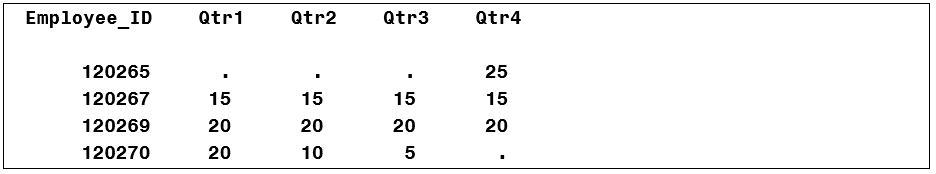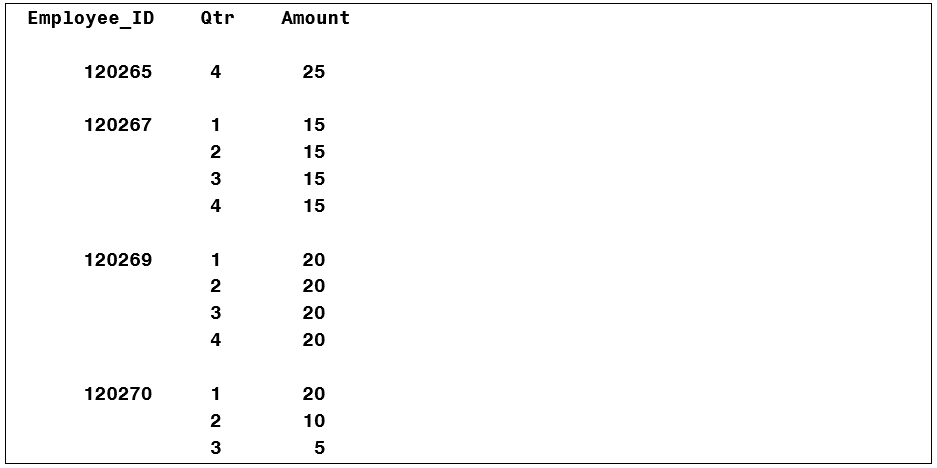Datasets can present themselves in different ways.

### A "Wide" Dataset### A "Tall" DatasetThe above datasets contain identical data, just arranged differently.

Which dataset is better? Generally, the tall dataset is better. Why?

The first advantage of the tall dataset is that it's ready-to-go for our statistical procedures (PROC MEANS, PROC FREQ, PROC TABULATE, PROC GCHART, etc.). For example, you can easily calculate:

• the average amount overall
• the total amount overall
• the average amount by quarter
• the total amount by quarter
• the average amount by employee
• the total amount by employee
• all of the above
• any combination of the above

Just use different combinations of class variables.  For example:

```proc means data=tall sum mean; var Amount; class Employee_ID Qtr; run;```

Statistical analysis and graphics are not so simple with the wide dataset.

A second advantage of the tall dataset is that it does not waste space storing missing values.

A third advantage of the tall dataset is that it imposes no artificial limit, such as 4, on the number of measures per employee, or per whatever. Suppose, instead of calendar quarters, the database stores information about each employee's dependents? Or health care visits? How many dependents, or visits, should we allow for? Four?  Ten? Anything you choose is arbitrary. With a tall dataset, if there is another dependent, or visit, you simply add another row. There are no missing values and no artificial limit.

If you have a wide dataset, how do you convert it to a tall dataset?

Easy, with some PROC TRANSPOSE tricks:

```proc transpose data=wide out=tall(where=(amount ne .) rename=(col1=Amount)) name=Qtr; by Employee_Id; run;```

SAS log:

```NOTE: There were 124 observations read from the data set WORK.WIDE. NOTE: The data set WORK.TALL has 417 observations and 3 variables.```

You can, of course, perform the same task with a datastep:

```data tall; keep Employee_ID Qtr Amount; array numbers{4} qtr1-qtr4; set wide; do Qtr=1 to 4; Amount=numbers{Qtr}; if Amount ne . then output; end; run;```

SAS log:

```NOTE: There were 124 observations read from the data set WORK.WIDE. NOTE: The data set WORK.TALL has 417 observations and 3 variables.```

The DATA step requires slightly more code than PROC TRANSPOSE, but you can see, and control, exactly what it's doing.

What if, notwithstanding the advantages of a tall dataset, you want to transpose from tall to wide? Again, you can use PROC TRANSPOSE:

```proc transpose data=tall out=wide(drop=_name_) prefix=Qtr; by Employee_ID; run;```

SAS log:

```NOTE: There were 417 observations read from the data set WORK.WIDE. NOTE: The data set WORK.TALL has 124 observations and 5 variables.```

Or the DATA step:

```data wide; keep Employee_ID qtr1-qtr4; retain qtr1-qtr4; array numbers{4} qtr1-qtr4; set tall; by Employee_ID; if first.Employee_ID then call missing(of qtr1-qtr4); numbers{Qtr}=Amount; if last.Employee_ID; run;```

SAS log:

```NOTE: There were 417 observations read from the data set WORK.WIDE. NOTE: The data set WORK.TALL has 124 observations and 5 variables.```

The technique you choose is a matter of personal preference.  SAS gives you choices!

Share